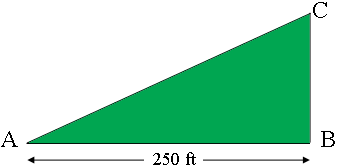SEARCH HOMEMath Central Quandaries & QueriesQuestion from Phyllis, a parent: What is the perimeter of a five acre triangle with one side being 250 feet and the second side would be at a 90 degree angle. Thank you.Hi Phyllis,

There are 43560 square feet in an acre so 5 acres is $5 \times 43560$ square feet.

Here is the triangle you describe.The area of a triangle can be expressed as $\large \frac12 \normalsize \mbox{ base } \times \mbox{ height.}$ Since the angle at $B$ is a right angle the length of the side from $B$ to $C$ is the height of the triangle. Solve for the height of the triangle. NOw you know the lengths of two sides of a right triangle so you can use Pythagoras Theorem to find the length of the third side.

PennyMath Central is supported by the University of Regina and the Imperial Oil Foundation.